# B.4 The Evolution Operator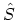To solve the equations of motion in the interaction picture (B.7), a unitary operator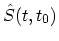that determines the state vector at time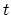in terms of the state vector at time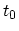is introduced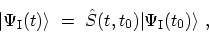(B.15)satisfies the initial condition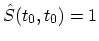. For finite timescan be constructed explicitly by employing the SCHRÖDINGER picture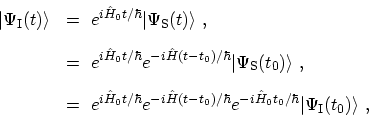(B.16)

which therefore identifies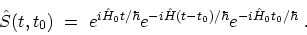(B.17)

Since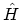and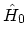do not commute with each other, the order of the operators must be carefully maintained. Equation (B.17) immediately yields several general properties of•, implying thatis unitary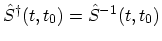,
•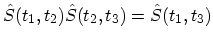, which shows thathas the group property, and
•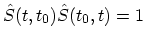, implying that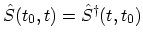.
Although (B.17) is the formal solution to the problem posed by (B.15), it is not very useful for computational purposes. Instead one can construct an integral equation for, which can then be solved by iteration. It follows from (B.7) and (B.15) thatsatisfies the differential equation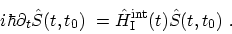(B.18)

Integrating both sides of the (B.18) with respect to time with the initial conditionyields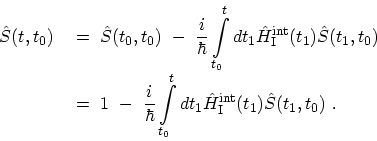(B.19)

By iterating this equation repeatedly one gets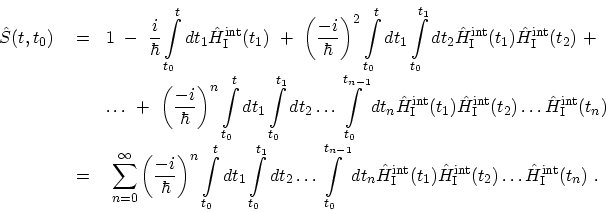(B.20)

Equation (B.20) has the characteristic feature that the operator containing the latest time stands farthest to the left. At this point it is convenient to introduce the time-ordering operator denoted by the symbol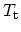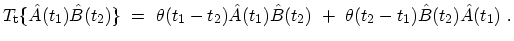(B.21)

where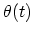is the step functionB.1. Each time two FERMIons are interchanged, the resulting expression changes its sign. By rearranging the integral using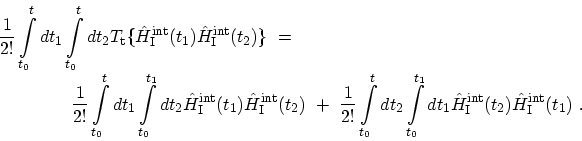(B.22)

The second term on the right hand-side is equal to the first, which is easy to see by just redefining the integration variables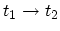,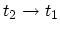. Thus one gets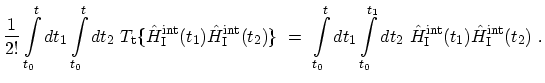(B.23)

Thus for the expansion of theone obtains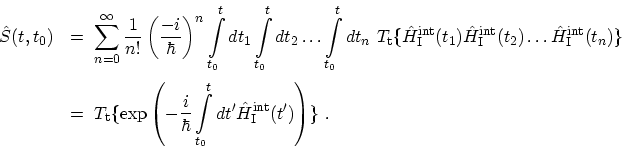(B.24)

M. Pourfath: Numerical Study of Quantum Transport in Carbon Nanotube-Based Transistors